R Programming tutorial

•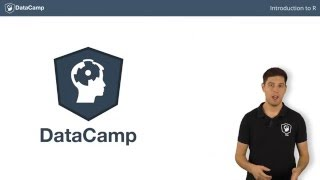WatchR tutorial - The True Basics of R
•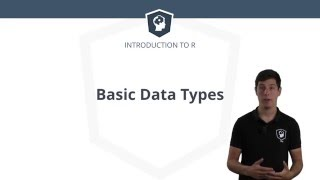WatchR tutorial: The basic data types in R
•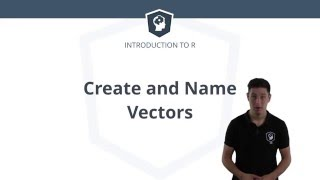WatchR tutorial - How to Create and Name Vectors in R
•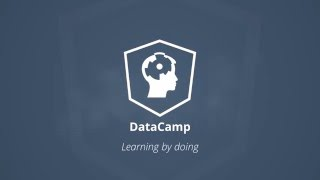WatchR tutorial - Different Ways to Create and Name Vectors in R
•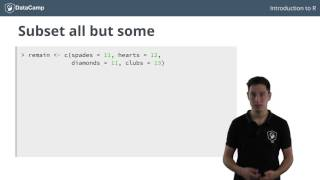WatchR tutorial - Subsetting your Vectors in R
•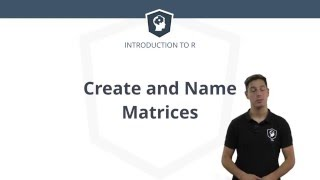WatchR tutorial - Learn How to Create and Name Matrices in R
•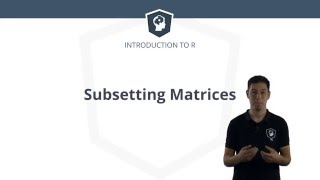WatchR tutorial - Learn How to Subset Matrices in R
•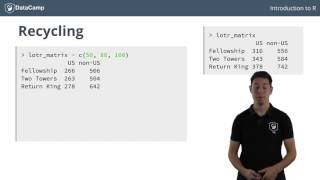WatchR tutorial - Matrix Arithmetic in R
•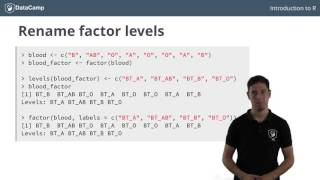WatchR tutorial - Using Factors in R
•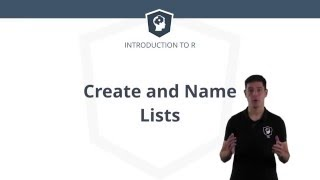WatchR tutorial - How to Create & Name Lists in R
•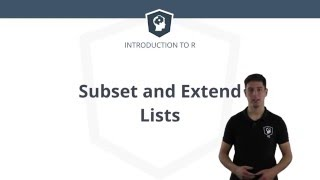WatchR Tutorial - How to Subset & Extend Lists in R
•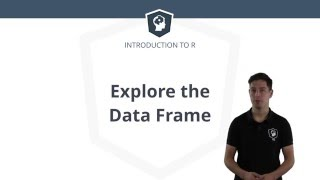WatchR Tutorial - Using the Data Frame in R
•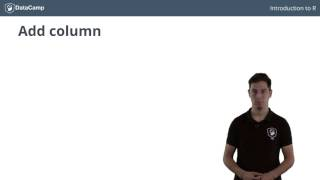WatchR tutorial - Learn How to Subset, Extend & Sort Data Frames in R
•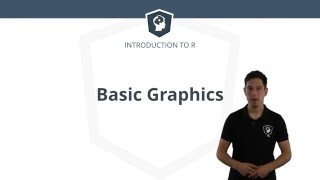WatchR Tutorial - Making Basic Graphics in R
•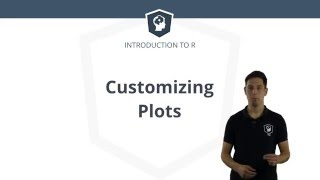WatchR Tutorial - Customizing Your Plots In R
•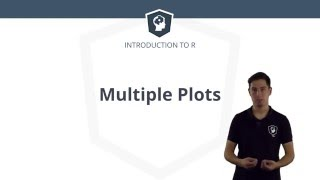WatchR Tutorial - How to plot multiple graphs in R
•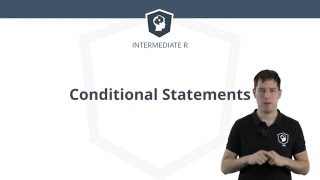WatchR Tutorial - How To Use Conditional Statements in R
•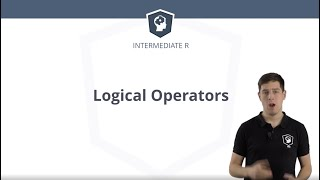WatchR Tutorial - Logical Operators and Vectors in R
•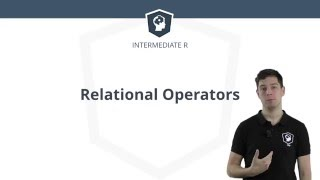WatchR Tutorial - Relational Operators in R
•WatchR tutorial: Writing functions in R
•WatchR tutorial: Scoping with R
•WatchR tutorial: Data structures in R
•WatchR tutorial: Working with for loops in R

Check out the r-tutorials instructor profile for more info. Let’s take a look at the content and how the course is structured: We will start with installation, the R and RStudio interface, add on packages, how to use the R exercise database and the R help tools. Then we will learn various ways to import data, first coding steps …